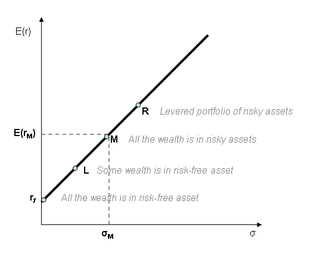# Capital Market Line - Explained

What is the Capital Market Line?

# What is the Capital Market Line (CML)?

Capital market line (CML) is a graph representing a portfolio's expected return based upon a given level of risk. On the horizontal axis is the portfolio standard deviation. On the vertical axis is the expected rate of return.Capital Market Line

L = Standard deviation of L portfolio

M = Standard deviation of market returns

R = Standard deviation of R Portfolio

## How does a Capital Market Line (CML) Work?

The CML is a special version of the Capital Allocation Line (CAL). The CAL demonstrates an efficient frontier for a portfolio of risky assets. The CML integrates a weighted percentage of risk-free assets. This makes the risk-return expectation linear, whereas the CAL is a curved frontier.

The equation for the CML is:

E(Rc) = y E(RM) + (1 y) RF

E(Rc) = the expected return of the portfolio

E(RM) = the expected return of the market portfolio

RF = the risk-free rate of return.

The line represents the risk premium that an investor earns when he or she takes on additional risk.

There is diversification of market portfolio that carries systematic risks, and whose expected return equals that of the entire market return.

Most people confuse the security market line (SML) with the capital market line (CML). We derive the security line from the capital market line. CML shows a specific portfolios rates of return while the SML represents a market risk as well as given times return. It also shows individual assets expected returns. CML represents the total risk, and its measurement is in the SML (beta or systematic risk). Fairly priced securities always plot on the SML and CML. Note that securities which generate higher results for a certain risk, are usually above the SML or CML, and are always underprice and vice versa.